## ML Aggarwal Class 10 Solutions for ICSE Maths Chapter 20 Heights and Distances MCQS

These Solutions are part of ML Aggarwal Class 10 Solutions for ICSE Maths. Here we have given ML Aggarwal Class 10 Solutions for ICSE Maths Chapter 20 Heights and Distances MCQS

More Exercises

Choose the correct answer from the given four options (1 to 9):

Question 1.
In the given figure, the length of BC is
(a) 2 √3 cm
(b) 3 √3 km
(c) 4 √3 cm
(d) 3 cm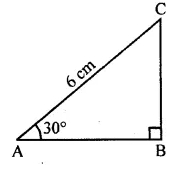Solution:
In the given figure, $$\\ \frac { BC }{ AC }$$ = sin 30°
⇒ $$\\ \frac { BC }{ 6 }$$ = $$\\ \frac { 1 }{ 2 }$$
⇒ BC = $$\\ \frac { 6 }{ 2 }$$ = 3cm (d)

Question 2.
In the given figure, if the angle of elevation is 60° and the distance AB = 10 √3 m, then the height of the tower is
(a) 20 √3 cm
(b) 10 m
(c) 30 m
(d) 30 √3 m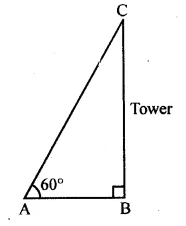Solution:
In the given figure,
∠A = 60°, AB = 10 √3 m
Let BC = h
tan 60° = $$\frac { h }{ 10\sqrt { 3 } }$$
⇒ $$\sqrt { 3 } =\frac { h }{ 10\sqrt { 3 } }$$
⇒ h = 10 √3 × √3 = 10 × 3 = 30 m (c)

Question 3.
If a kite is flying at a height of 40 √3 metres from the level-ground, attached to a string inclined at 60° to the horizontal, then the length of the string is
(a) 80 m
(b) 60 √3 m
(c) 80 √3 m
(d) 120 m
Solution:
Let K is kite
Height of KT = 40 √3 m
Angle of elevation of string at the ground = 60°
Let length of string AK = x mQuestion 4.
The top of a broken tree has its top touching the ground (shown in the given figure) at a distance of 10 m from the bottom. If the angle made by the broken part with ground is 30°, then the length of the broken part is
(a) 10 √3 m
(b) $$\frac { 20 }{ \sqrt { 3 } }$$
(c) 20 m
(d) 20 √3 mSolution:
From the figure, AC is the height of tree and from B, it was broken
AB = A’C
Angle of elevation = 30°
A’C = 10 m
Let AC = hm’
and A’B = x m
BC = h – x m
$$cos\theta =\frac { A’C }{ A’B }$$
$$cos{ 30 }^{ o }=\frac { 10 }{ x }$$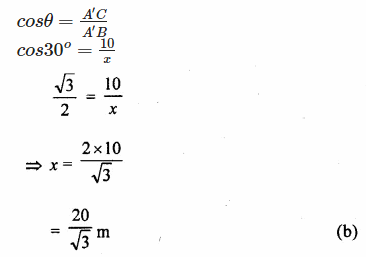Question 5.
If the angle of depression of an object from a 75 m high tower is 30°, then the distance of the object from the tower is
(a) 25 √3 m
(b) 50√ 3 m
(c) 75 √3 m
(d) 150 m
Solution:
Height tower AB = 75 m
C is an object on the ground and angle of depression from A is 30°.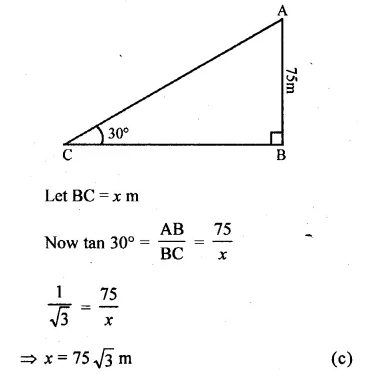Question 6.
A ladder 14 m long rests against a wall. If the foot of the ladder is 7 m from the wall, then the angle of elevation is
(a) 15°
(b) 30°
(c) 45°
(d) 60°
Solution:
Length of a ladder AB = 14 m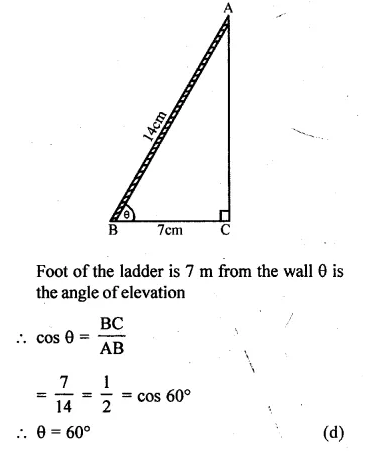Question 7.
If a pole 6 m high casts shadow 2 √3 m long on the ground, then the sun’s elevation is
(a) 60°
(b) 45°
(c) 30°
(d) 90°
Solution:
Height of pole AB = 6 m
and its shadow BC = 2√3 m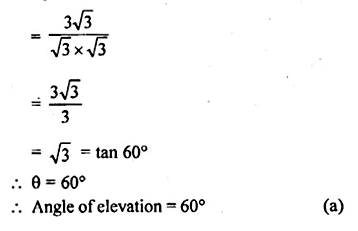Question 8.
If the length of the shadow of a tower is √3 times that of its height, then the angle of elevation of the sun is
(a) 15°
(b) 30°
(c) 45°
(d) 60°
Solution:
Let height of a tower AB = h m
Then its shadow BC = √3 hmQuestion 9.
In ∆ABC, ∠A = 30° and ∠B = 90°. If AC = 8 cm, then its area is
(a) 16 √3 cm²
(b) 16 m²
(c) 8 √3 cm²
(d) 6 √3 cm²
Solution:
In ∆ABC, ∠A = 30°, ∠B = 90°
AC = 8 cm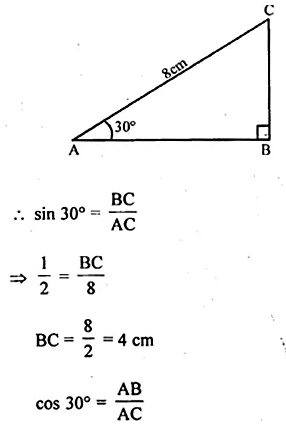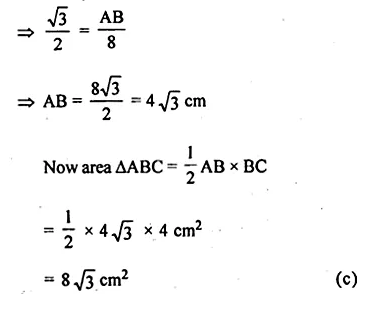We hope the ML Aggarwal Class 10 Solutions for ICSE Maths Chapter 20 Heights and Distances MCQS help you. If you have any query regarding ML Aggarwal Class 10 Solutions for ICSE Maths Chapter 20 Heights and Distances MCQS, drop a comment below and we will get back to you at the earliest.